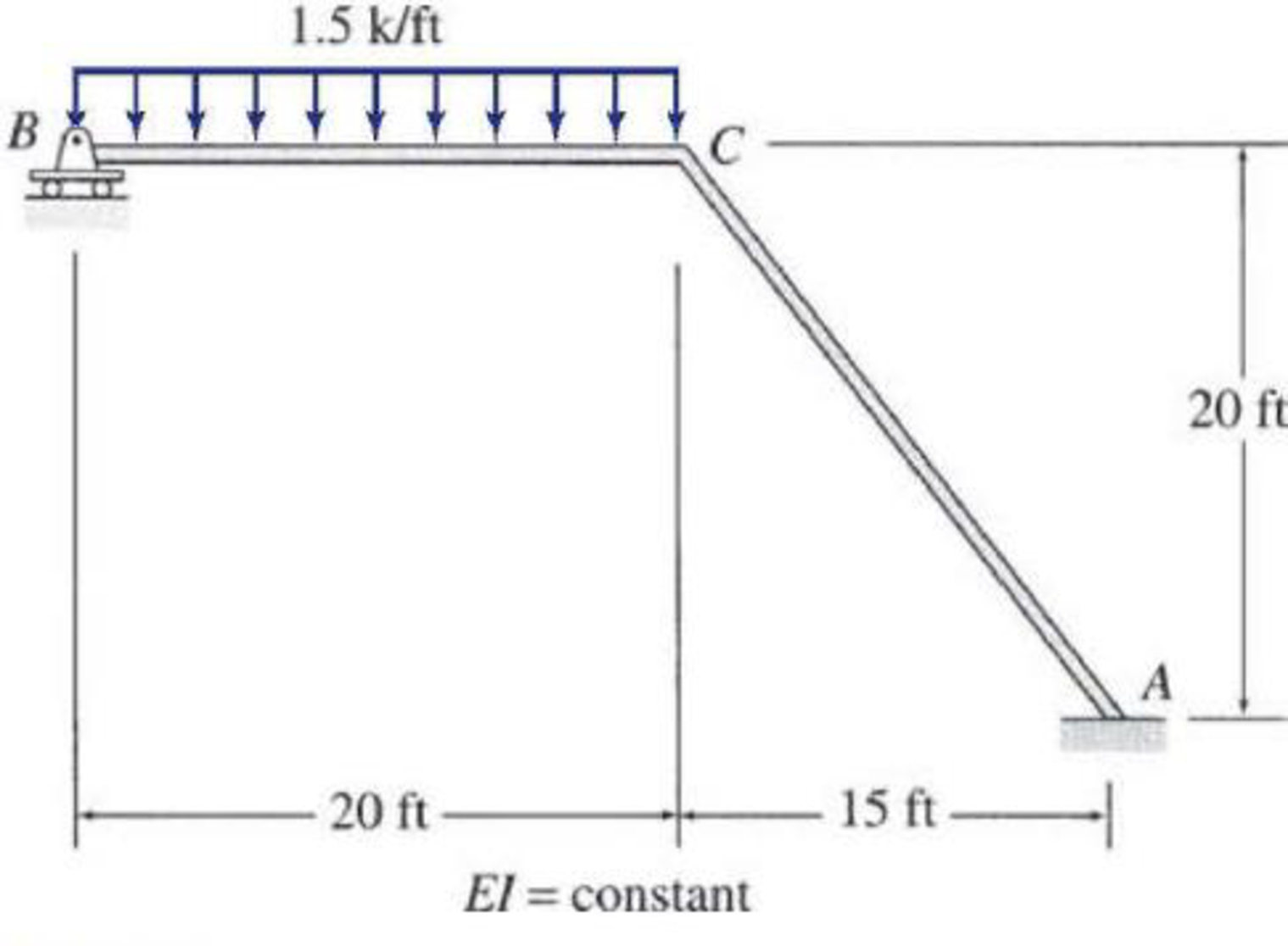# 15.24 through 15.31 Determine the member end moments and reactions for the frames shown in Figs. P15.24–P15.31 by using the slope-deflection method. FIG. P15.28

#### Solutions

Chapter
Section
Chapter 15, Problem 28P
Textbook Problem
69 views

## 15.24 through 15.31 Determine the member end moments and reactions for the frames shown in Figs. P15.24–P15.31 by using the slope-deflection method.FIG. P15.28To determine

Find the member end moments and reaction for the frames.

### Explanation of Solution

Fixed end moment:

Formula to calculate the fixed moment for UDL is WL212.

Calculation:

Consider the flexural rigidity EI of the frame is constant.

Show the free body diagram of the entire frame as in Figure 1.

Refer Figure 1,

Calculate the fixed end moment for BC.

FEMBC=1.5×20212=50kft

Calculate the fixed end moment for CB.

FEMCB=50kft

Calculate the fixed end moment for AC.

FEMAC=0kft

Calculate the fixed end moment for CA.

FEMCA=0kft

Chord rotations:

Show the free body diagram of the chord rotation of the frame as in Figure 2.

Calculate the length of AC by using Pythagoras theorem.

LAC=(202)+(152)=25ft

Calculate the chord rotation of the frame BC.

ψBC=C1CLBC=34Δ20=0.0375Δ

Calculate the chord rotation of the frame AC.

ψAC=CCLAC=54Δ25=0.05Δ

Calculate the slope deflection equation for the member AC.

MAC=2EIL(2θA+θC3ψAC)+FEMAC

Substitute 25 ft for L, 0 for θA, 0.05Δ for ψAC and 0 for FEMAC.

MAC=2EI25(2(0)+θC3(0.05Δ))+0=0.08EIθC0.012Δ        (1)

Calculate the slope deflection equation for the member CA.

MCA=2EIL(2θC+θA3ψCA)+FEMCA

Substitute 25 ft for L, 0 for θA, 0.05Δ for ψCA, and 0 for FEMCA.

MCA=2EI25(2θC+03(0.05Δ))+0=0.16EIθC0.012Δ        (2)

Calculate the slope deflection equation for the member CB.

MCB=3EIL(2θB+θCψCB)+FEMCB+FEMCB2

Substitute 20 ft for L, 0 for θB, 0.0375Δ for ψCB, and 50kft for FEMCB.

MCB=3EI20(2(0)+θC(0.0375Δ))50502=0.15EIθC+0.005625EIΔ75        (3)

Calculate the slope deflection equation for the member BC.

MBC=0

Write the equilibrium equation as below.

MCA+MCB=0

Substitute equation (2) and equation (3) in above equation.

0.16EIθC0.012Δ+0.15EIθC+0.005625EIΔ75=00.31EIθC0.006375EIΔ=75        (4)

Show the free body diagram of the entire frame due to sway force as in Figure 3.

Show the free body diagram of the member AC due to sway force as in Figure 4.

Calculate the horizontal reaction at the member AC due to sway force by taking moment about point A.

MA=0(SAC×25)+MAC+MCA=0SAC=MAC+MCA25

Calculate the reaction of the support C due to sway force by taking the moment about O

### Still sussing out bartleby?

Check out a sample textbook solution.

See a sample solution

#### The Solution to Your Study Problems

Bartleby provides explanations to thousands of textbook problems written by our experts, many with advanced degrees!

Get Started

Find more solutions based on key concepts
What is the difference between a dimension and unit?

Engineering Fundamentals: An Introduction to Engineering (MindTap Course List)

If the C.I.A. triad is incomplete, why is it so commonly used in security?

Principles of Information Security (MindTap Course List)

What is object-oriented analysis, and what e some advantages of this method?

Systems Analysis and Design (Shelly Cashman Series) (MindTap Course List)

List cloud computing resources. Describe how businesses use cloud computing to manage resources.

Enhanced Discovering Computers 2017 (Shelly Cashman Series) (MindTap Course List)

When facing, why should the tool not be fed past the center of the workpiece?

Precision Machining Technology (MindTap Course List)

If your motherboard supports ECC DDR3 memory, can you substitute non-ECC DDR3 memory?

A+ Guide to Hardware (Standalone Book) (MindTap Course List)

What characteristics of the weld bead do the weaving of the electrode cause?

Welding: Principles and Applications (MindTap Course List)